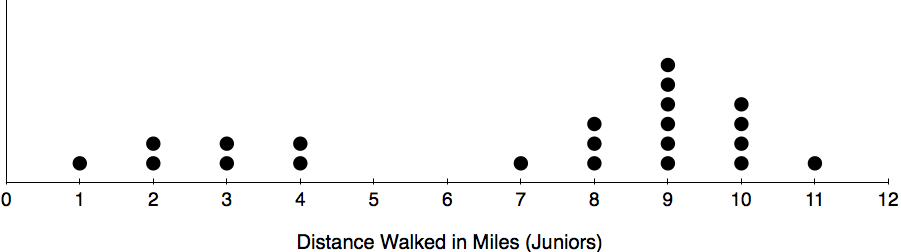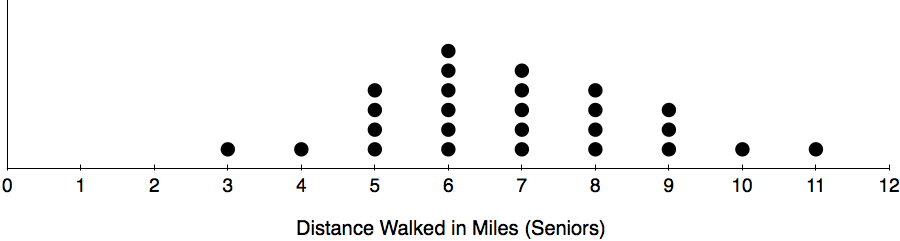# Descriptive Statistics

## Objective

Calculate and interpret the spread (variance) of a data set.

## Common Core Standards

### Core Standards

?

• HSS-ID.A.3 — Interpret differences in shape, center, and spread in the context of the data sets, accounting for possible effects of extreme data points (outliers).

• HSS-ID.A.4 — Use the mean and standard deviation of a data set to fit it to a normal distribution and to estimate population percentages. Recognize that there are data sets for which such a procedure is not appropriate. Use calculators, spreadsheets, and tables to estimate areas under the normal curve.

?

• 7.SP.B.3

• 7.SP.B.4

## Criteria for Success

?

1. Describe the spread of a data set using the visual spread in comparison to another data set, and the variability of the data set as how the data set varies from the mean.
2. Quantify variability and spread generally by the overall range of the data set.
3. Describe that the best way to further quantify the spread of a data set is based on the measure of center most appropriate for the data set. Median and interquartile range are outlier resistant so are best for skew distributions. Mean and standard deviation are best for symmetrical distributions.
4. Calculate the deviation of each data item from the mean. Describe that larger deviations indicate that the item is more spread from the data item.
5. Use a calculator to find the variance, and describe this as the measure of the average of the squared differences of each term from the mean.
6. Describe that the less variance a data set has, the more closely the data is centered around the mean and the more consistent the data is.

## Tips for Teachers

?

• This lesson moves from conceptual understanding of spread to calculating variability to summing this up with the idea of variability as a measure of consistency.
• Grade 7 calculates the MAD (mean absolute deviation). We won’t go back to that concept, but you should be familiar with it.
• Read Chapter 3 of What Is a p-Value Anyway? Andrew J. Vickers, Pearson (2009).

## Anchor Problems

?

### Problem 1

Given data set: 4, 2, 6, 3, 9, 2, 1, 3, 4
How far is the data from the mean? How would you figure this out?

### Problem 2

Which of the following data sets shows more consistency? How do you know?#### References

EngageNY Mathematics Algebra I > Module 2 > Topic A > Lesson 3Exercise 8

Algebra I > Module 2 > Topic A > Lesson 3 of the New York State Common Core Mathematics Curriculum from EngageNY and Great Minds. © 2015 Great Minds. Licensed by EngageNY of the New York State Education Department under the CC BY-NC-SA 3.0 US license. Accessed Dec. 2, 2016, 5:15 p.m..

## Problem Set

?

The following resources include problems and activities aligned to the objective of the lesson that can be used to create your own problem set.

• Use the EngageNY Problem Set for practice (for the deviations section).
• Use the 6th grade EngageNY Lesson 14 and Lesson 15 Problem Set for practice, but add in questions to find the spread of the data.